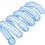# Deriving Newton's 1st Law for One-Dimensional Motion

Here is my attempt at using the classical least-action principle to derive Newton's 1st law of motion for straight-line motion.

Suppose a particle goes from $x = 0$ to $x = D$ between time $t_1$ and time $t_2$. Suppose also that space is potential-free (and thus force-free). The action is defined as:

$S = \int_{t_1}^{t_2} ( E - U) \, dt = \int_{t_1}^{t_2} E \, dt$

Suppose we discretize the path into $N$ constant-velocity periods. $N$ can be made arbitrarily large. For each period, the velocity is $v_k$. The action becomes:

$S = \frac{m}{2} \, \Sigma_{k=1}^{k=N} v_k ^2 \, \Delta t \\ \Delta t = \frac{t_2 - t_1}{N}$

The actual path must minimize the action while satisfying the constraint that the changes in $x$ position must sum to $+D$. We can use the method of Lagrange multipliers with the following Lagrangian:

$L = \frac{m}{2} \, (\Sigma_{k=1}^{k=N} v_k ^2 \, \Delta t) + \lambda \Big ((\Sigma_{k=1}^{k=N} v_k \, \Delta t) - D \Big)$

Taking partial derivatives with respect to the period-specific velocities and setting to zero (per standard practice) results in:

$\frac{\partial{L}}{\partial{v_1}} = m \, v_1 \, \Delta t + \lambda \, \Delta t = 0 \implies v_1 = -\frac{\lambda}{m} \\ \frac{\partial{L}}{\partial{v_2}} = m \, v_2 \, \Delta t + \lambda \, \Delta t = 0 \implies v_2 = -\frac{\lambda}{m} \\ \text{etc.}$

Thus, for motion along a straight line in potential-free (force-free) space, the least-action principle dictates that the velocity must be constant.Note by Steven Chase
2 years, 1 month ago

This discussion board is a place to discuss our Daily Challenges and the math and science related to those challenges. Explanations are more than just a solution — they should explain the steps and thinking strategies that you used to obtain the solution. Comments should further the discussion of math and science.

When posting on Brilliant:

• Use the emojis to react to an explanation, whether you're congratulating a job well done , or just really confused .
• Ask specific questions about the challenge or the steps in somebody's explanation. Well-posed questions can add a lot to the discussion, but posting "I don't understand!" doesn't help anyone.
• Try to contribute something new to the discussion, whether it is an extension, generalization or other idea related to the challenge.

MarkdownAppears as
*italics* or _italics_ italics
**bold** or __bold__ bold
- bulleted- list
• bulleted
• list
1. numbered2. list
1. numbered
2. list
Note: you must add a full line of space before and after lists for them to show up correctly
paragraph 1paragraph 2

paragraph 1

paragraph 2

[example link](https://brilliant.org)example link
> This is a quote
This is a quote
    # I indented these lines
# 4 spaces, and now they show
# up as a code block.

print "hello world"
# I indented these lines
# 4 spaces, and now they show
# up as a code block.

print "hello world"
MathAppears as
Remember to wrap math in $$ ... $$ or $ ... $ to ensure proper formatting.
2 \times 3 $2 \times 3$
2^{34} $2^{34}$
a_{i-1} $a_{i-1}$
\frac{2}{3} $\frac{2}{3}$
\sqrt{2} $\sqrt{2}$
\sum_{i=1}^3 $\sum_{i=1}^3$
\sin \theta $\sin \theta$
\boxed{123} $\boxed{123}$

Sort by:

I like how you have approached a problem involving the calculus of variations by discretizing the integral. In addition to proving that velocity remains constant, another important principle can be drawn attention to, using this analysis. That being the principle of conservation of momentum. On inspecting the Lagrange multiplier $\lambda$, one can see that it can be interpreted as linear momentum which is a constant. This validates the notion that momentum is conserved in the absence of external forces, which is an inference usually made using Newton's second law of motion.

An alternate approach to this proof would be to avoid discretization of the integral altogether. The action $S$ becomes minimum when Euler - Lagrange's equation is satisfied. This is based on the Calculus of variations.This would lead to:

$\frac{d}{dt}\frac{\partial{E}}{\partial{\dot{x}}} - \frac{\partial{E}}{\partial{x}} = 0$

Now, using the fact that in 1-D motion, $E = \frac{1}{2}m\dot{x}^2$, this can be plugged into Lagrange's equation giving us: $m\ddot{x} = 0$. Integrating this leads to:

$m\dot{x} = constant$

Which is the desired result.

An interested reader can delve into the concepts of calculus of variations. Wikipedia is a good starting point.

- 2 years, 1 month ago

@Karan Chatrath Do you believe this line of argument?

- 2 years, 1 month ago

Good point about the lambdas. I had neglected to consider their physical meaning since I was only using them as a bridge to equate velocities from different infinitesimal periods. And the first law does indeed come effortlessly from Lagrangian Mechanics, once LM has been established as a valid principle. I have become proficient in the application of LM to dynamics problems, but I am not yet very familiar with its derivation from variational calculus (that will be some homework). The discretized approach appeals to me on an intuitive level. On that subject, I have posted a three-dimensional version at the link below, which should easily extend to any number of dimensions.

Thanks